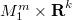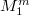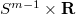﻿

### Homogeneous Manifolds in Codimension Two Revisited

#### Abstract

In this paper we study Riemannian homogeneous submanifolds of  Euclidean spaces in codimension two. If the index of relative  nullity of the second fundamental form is relatively low, we prove  that the submanifold is a product$M_1^{m} \times {\bf R}^k$ where$M_1^{m}$ is either isometric to a sphere or to a compact  isoparametric hypersurface of the sphere or covered by$S^{m-1} \times {\bf R}$. For homogeneous Einstein manifolds we obtain a  complete classification which improves the result in .

DOI Code: 10.1285/i15900932v21n1p49

Keywords: Relative nullity; Rigid immersions; Isometry; Einstein manifolds

Classification: 53C40; 53C42

Full Text: PDF